This video was brought to you by GeneralPAC.com, making power systems Intuitive, Open and Free for Everyone, Everywhere. Consider subscribing and supporting through patreon.com/GeneralPAC. This is a mechanism for you to support us financially so we can continue making high quality power system video tutorials. Our corporate sponsor for this topic is AllumiaX.com from Seattle, Washington. Contact them for industrial and commercial power system studies.

The three phase transformer WYE-WYE connection and the introduction to the infamous square root 3, module 4 – Evaluating the Line to Line equation.

Be sure to check out the resources section under this video for helpful comments, suggestions and clarifications.

In the last couple of videos, we took a simplified approach to 3 phase transformers Wye-Wye connections and current and voltage quantities.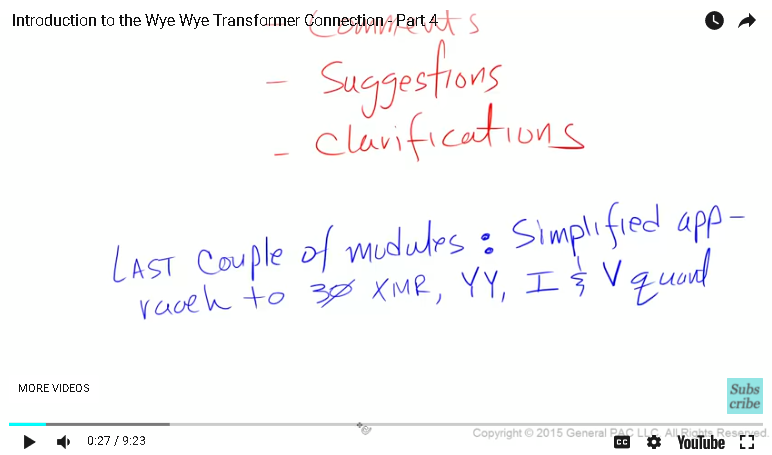In this module, we are going to talk thoroughly about the line to line voltage and phase voltage relationship.

Okay, so we understand the particular arrangement of Wye connected transformer. The line to line voltage between line A and line B is equal to the primary phase A voltage minus the primary phase B voltage.

The question is, how would we evaluate this equation. Remember, this is where the Root 3 comes from.

Our next step is to define our phase voltages. If you have doubts, when I was learning this, I too have doubts here.

Let’s play a close attention and learn this stuff intuitively.

The root 3 comes from the fact that we have a balanced system that is typically running in a normal operation or steady-state. All three terminologies pretty much the same thing.

A balanced system is a system, which maintains 3 key rules at all times. These rules are specifically for current and voltage quantities.

Note, the balanced system is methodically introduced in a different module. Be sure to check out the resources section for additional information.

The three rules for the balanced system are,
Rule no 1: Phase A, B and C voltages or currents must have equal magnitudes.
Rule no 2: Phase A, B and C voltages or currents must have 120 degree phase displacement between each other.

And rule no 3: Phase A, B and C voltages or currents must have either in ABC or ACB phase rotation or phase sequence & must rotate in a counter clockwise direction.

Okay, so we are going to create a balanced set of phasors and then verify the following all 3 rules in a balanced system.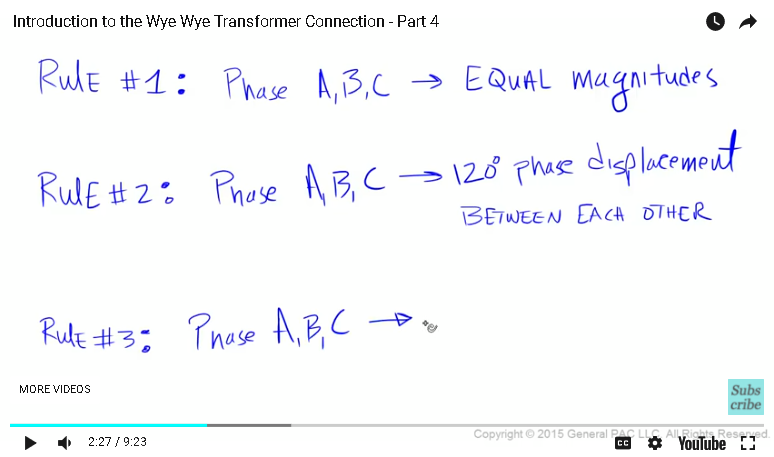The first thing, that we will do is to draw a phasor diagram with an X-axis and a Y-axis.

We are going to draw a nice round circle, right in the middle. We are also going to label the degree marks at every 30 degrees’ increments.

We are also draw a reference line in a different colour at the zero degree mark.

Let’s assume our phase A voltage is 2400 volts at 0 degrees, our phase B voltage is 2400 volts at 240 degrees and our phase C voltage is 2400 volts at a 120 degrees.

Is this a balanced set of voltages?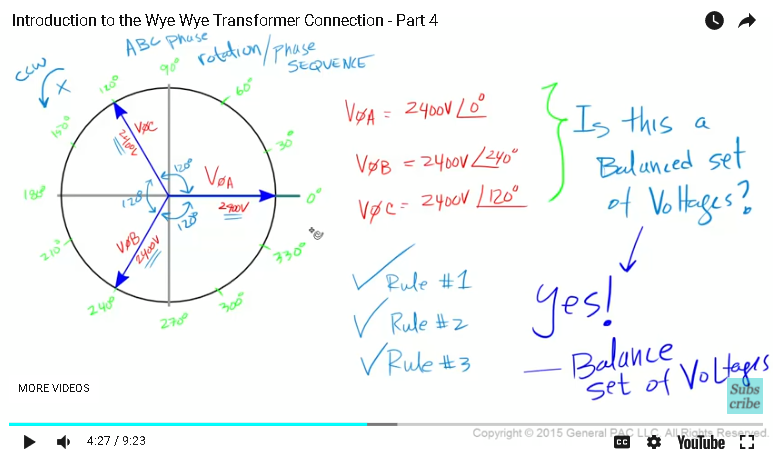Well, let’s see, we have equal magnitude of 2400 volts for phase A, B and C voltages, so we follow rule no 1.

We have a 120 degrees phase displacement between all 3 phasors, so we follow rule no 2.

We are assuming that all 3 phasors are rotating in a counter clockwise direction and while they rotate, the three phasors produce an ABC phase rotation or phase sequence following rule no 3.

And this my friends, we call the balanced set of three phase voltages, which contribute to something we called a balanced system.

So the next question is what we do with a balanced set of voltages. We evaluate our equations off course.

The following are the equations, we developed in the last couple of modules.

Okay, so we are going to do this in three simple steps.

Step no 1: okay the first step is to draw a phasor diagram similar to the previous one with phase A, B and C voltages in a balanced state rotating in a counter clockwise direction.

Remember that the magnitude of all three phasors is 2400 volts. This is what we are assuming, okay and this is the voltage across the primary windings of a transformer, so we are assuming that we have a 2400 volts across winding A on the primary side, 2400 volts across winding B on the primary side and 2400 volts across winding C on the primary side.

All 3 voltages are displaced by 120 degrees.

Step no 2: in the second step, we are going to add phase A voltage with phase B voltage, using the head to tail method.

So we will pick up a phase A and connecting to the head of Phase a, so essentially, the head of phase A is connected to the tail of phase B, hence the name, “The Head to Tail method”.

The resultant vector is the vector that starts at the origin and ends at the last phasor, which is the phase B voltage. So this is the resultant vector. But this resultant vector represents equal phase A voltage plus Phase B voltage.

However, the equation, we need to evaluate the is phase A voltage minus the phase B voltage. Since there is a minus sign associated with a phase B voltage, we need to take phase B voltage and rotated a 180 degrees.

It’s very important to keep in mind that 180 degree rotation is due to the minus sign in front of phase B voltage.

Okay, so we have phase A voltage, and we have the negative phase B voltage, we are going to perform the head to tail method.

So, we are going to take the tail of the negative phase B voltage and connected to the head of phase A voltage.

Step no 3: so the third step, we are going to draw the resultant vector. We will start at the origin and end over here.

The resultant vector equal phase A voltage minus phase B voltage.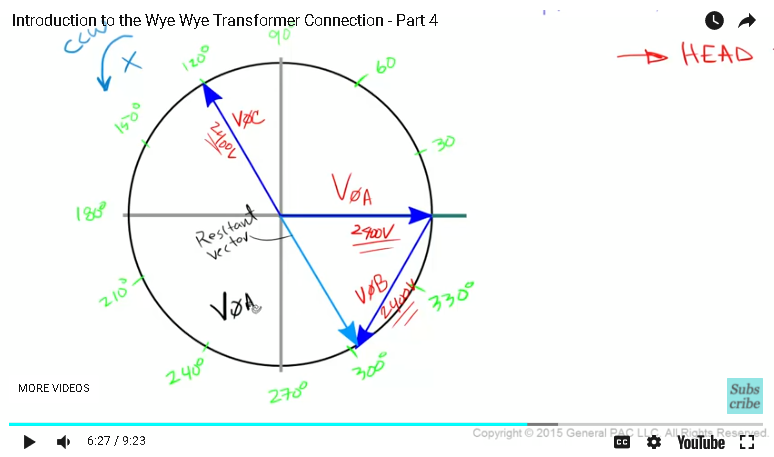Any idea, what we should call this resultant vector? How about VLL, AB. Remember our equation, the line to line voltage between line A and line B voltage is equal to the phase A voltage minus the phase B voltage.

The phasor diagram that we have drawn actually represents this particular equation particularly.

Let’s make some observation about our phasor diagram. The magnitude of VLL, AB is larger than phase A voltage, its also larger than phase B voltage.

The angle of VLL, AB is not the same as the phase A voltage or the phase B voltage.

So here is a question, how much larger in terms of VLL, AB compared to the magnitude of phase A, or the magnitude of phase B and those of us who answered, well its root 3 times larger or 173% larger and that my friends would be correct.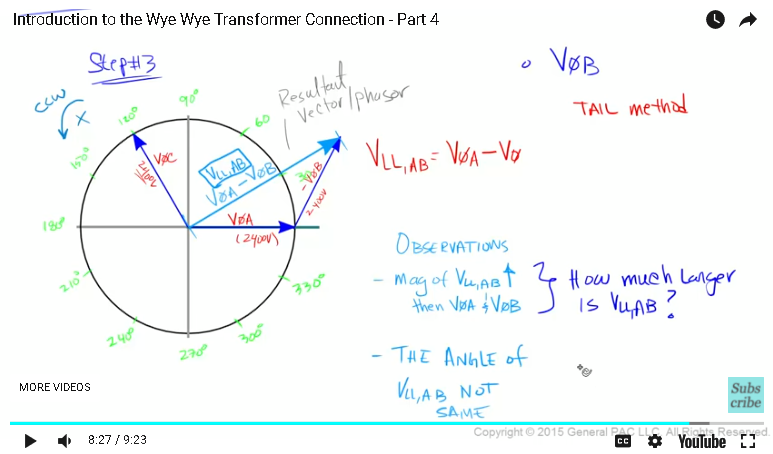When looking at the magnitudes alone. We should expect that the line to line voltage is equal to root 3 times larger than any phase voltage under the balanced set of voltages assumption.

We will continue our discussion about the root 3 in the next module.

Thank you. This module was brought to you by GeneralPac.com….making Power Systems Intuitive.

#### Greetings from the GeneralPAC Team!

We make high-quality Power Systems Video Tutorials on complex topics that are free and open to everyone!  Thank you so much for supporting us through Patreon so we can continue doing good and valuable work.

What is Patreon and why do we use it?

Patreon is a fantastic portal that allows our fans and community to make monthly contribution (like Netflix subscription) so we can continue creating high-quality power systems video tutorials. In return, you get access to incredible perks like voting on future topics, getting your questions answered, access to VIP Q/A webinars with the creators of GeneralPAC, and much more! We THANK YOU for supporting us

Why do we need your support?

An incredible amount of time and effort is needed to develop high-quality video tutorials. Each video (Part 1 for example) takes approximately 10 hours to complete which includes learning the concept ourselves, brainstorming creative ways to teach and explain the concepts, writing the script, audio recording, video recording, and editing. It's no wonder why Hundreds-of-Thousands of people have watched, liked, subscribed, and left positive comments on Youtube channel. Your support truly makes all the difference.